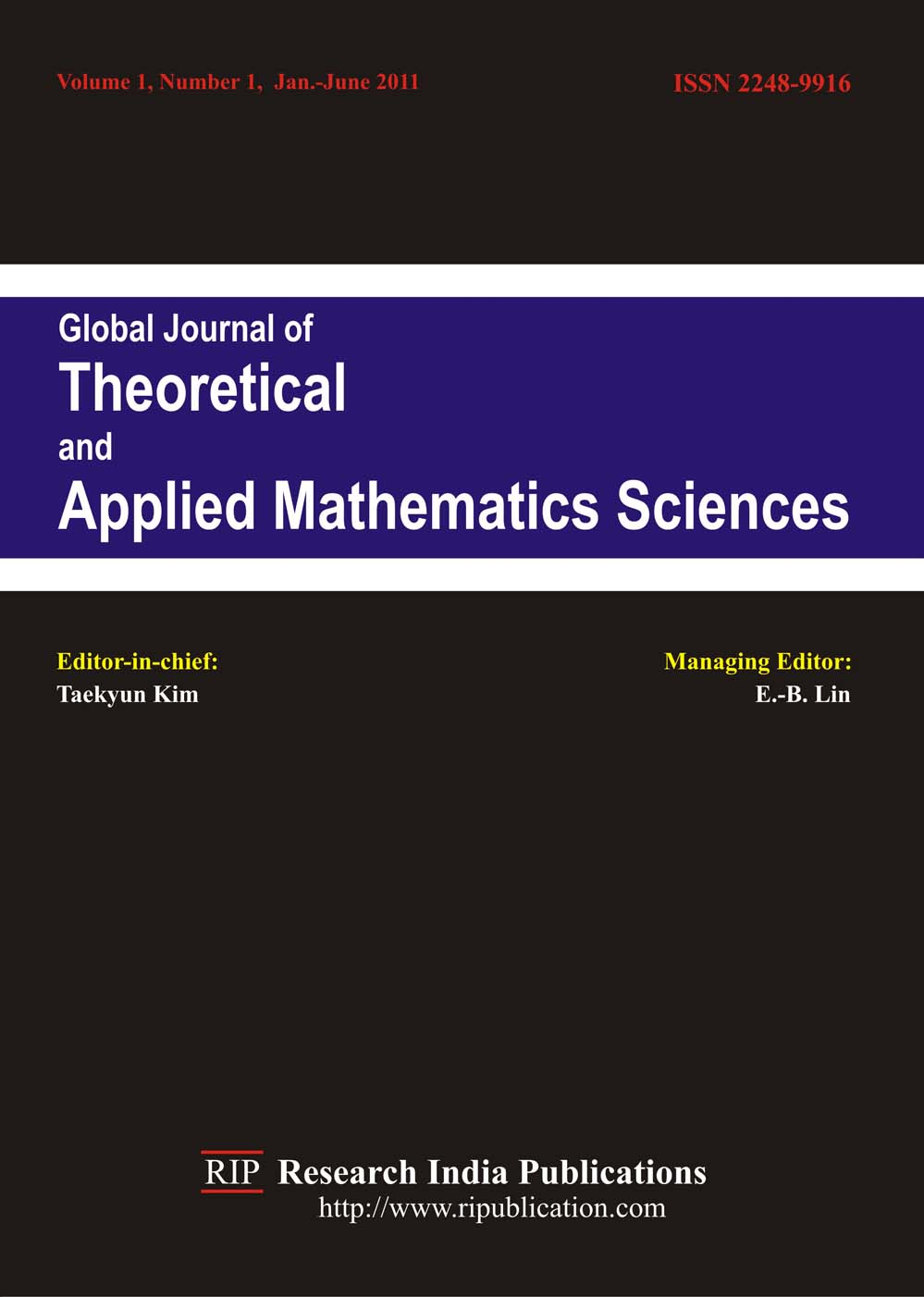Global Journal of Theoretical and Applied Mathematical Sciences (GJTAMS)

ISSN : 2248-9916

Editor-in-chief:Kahar El-Hussein,

Mathematics Department,

Al-Jouf University,

Al-Jouf, Kingdom of Saudi Arabia

Aims and Scope: The Global Journal of Theoretical and Applied Mathematical Sciences (GJTAMS) is an international journal of high quality devoted to the publication of original research papers from pure and applied mathematics with some emphasis on all areas and subareas of mathematical analysis and their broad range of applications. Areas and subareas of interest include (but are not limited to) approximation theory; statistics; probability; fluid mechanics; Fuzzy mathematics; transport theory; mathematical biology, including population dynamics; wave propagation; special functions; algebra and applications; combinatorics; coding theory; fractional analysis; solid mechanics; variational methods; financial mathematics; asymptotic methods; graph theory; fractals; moment theory; scattering theory; number theory and applications; geometry of Banach spaces; topology and applications; complex analysis; stochastic process; bifurcation theory; differential equations; difference equations; dynamical systems; functional differential equations; numerical analysis; partial differential equations; integral equations; operator theory; Fourier analysis; matrix theory; semigroups of operators; mathematical physics; convex analysis; applied harmonic analysis; optimization; wavelets; signal theory; ultrametric analysis; optimal control; fixed-point theory and applications; reaction-diffusion problems, discrete mathematics; automata theory and more....

Submission: Authors are requested to submit their papers electronically to submit@ripublication.com with mention journal title (GJTAMS) in subject line.

Frequency: Two issues per year.

Annual Subscription Price for year 2017:

Library/ Institutional: Print : US\$580.00 Online Only: US\$560.00

Print + Online : US\$620.00

Individual/ Personnel: Print  US\$290.00

Inside India: Rs.3500.00Editorial BoardInstruction for AuthorsVol. 1 No.1   No.2    No.3   (2011)Vol. 2 No.1   No. 2   No. 3  (2012)Vol. 3 No.1  (2013)Vol. 5 No.1  (2014)Vol. 6 No.1 No.2 (2016)Vol. 7 No.1 (2017)© All rights are reserved with Research India Publications                             Home  Journals  Contact